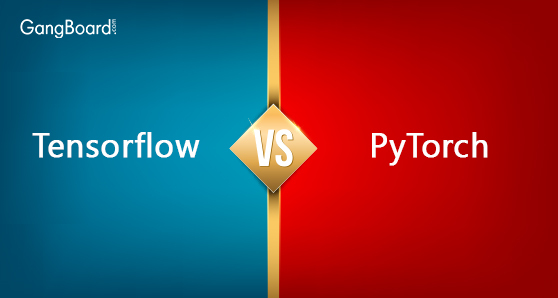# Tensorflow Vs PyTorch

May 20th, 2019

## Tensorflow Vs PyTorch

Are you using any of these frameworks? Who did not have listened about the comparison between PyTorch and Tensorflow? The faster search will show you the deep and clear intensity of these frameworks. There is a high probability of defending the framework which you believe in it. Like the core, these also are fuelled by the similar features of these two frameworks.
These two frameworks are having libraries which are open source having high-performance computation and provide support by a bigger company of techs such as facebook and google.
These two frameworks have the active and strong supporting community which are totally python based. These frameworks use the graphs for representing the operations and data flow and documented finely.
Taking all of these features into the account which we can predict that many things can be developed in one of these frameworks at the same cost. Thus the question arises that which of the framework can you use easily? And What is the main key difference between these frameworks?
On some data, we are researching that the community of developer for tracking the trends and assuming the future world of different sectors of technology. For the machine learning or data science in some particular, this clash is important. The framework will make a large and big impact on the path where the community of machine learning will take many years to occur.

While keeping this in mind, that we asked that the developers’ team who said that they are engaged in the machine learning or data science where these two frameworks used and how to use it. Tensorflow is nowadays a winner but PyTorch is playing on the same scores.
In total 3000 developers, it was found that more than 43 percents of developers use these two frameworks. But 43 percent is not divided equally as Tensorflow is around 3.4 times higher than PyTorch. In the total of 86% of data science or machine, learning developers said that they are now using Tensorflow, whereas only 11% were using PyTorch.
PyTorch has around more than 50% of its community which is also using the framework known as Tensorflow. Only 15% of the community of Tensorflow also uses a framework known as PyTorch somewhere. It would seem such as Tensorflow which is the must but PyTorch is also nice to use.

#### There are some things which really is different from all of the rest below

Tensorflow is used in the production and mostly integrated into the cloud which is seen by the bigger experience of the backend of Tensorflow users. In comparison to the ML developers, software architects and programmers inside the company are likely to the focus of Google on deployment via API like serving of Tensorflow which has now become the inspiration of it for many of the developers and they are now trying to forward the products of data into the environment.
And Pytorch is also used more than the framework of Tensorflow in the field of data analysis and models of ad-hoc in the context of business. In the community of PyTorch, there are many developers of a python who works on web applications. The versatility of such frameworks enable all researchers to test more ideas with the friction of zero and thus, it is the framework for the most advanced edge corner of cutting solutions.

### Difference Between Tensorflow and PyTorch

 TensorFlow PyTorch Tensorflow is totally based on Theano and is discovered by Google. PyTorch is totally based on Torch and is discovered by facebook. Tensorflow builts the static graph PyTorch creates the dynamic graph In this, you have to first define the whole graph of computation and then run into the model of machine learning. In this, you have to define or edit your graph which is then helpful using the variable length in RNNs. It creates a steeper learning curve than PyTorch where you have to learn more for its working sessions. PyTorch is pythonic in nature and develops the models of machine learning where it is hard for learning Tensorflow compared to PyTorch. It has a larger community with easy to determine resources and find out the solutions. PyTorch is a very new framework in terms of resources and so more content is found in Tensorflow compared to PyTorch. TensorBoard is the tools which allow visualization of models of machine learning in your browser directly. It does not have the tools, but you can use tools such as Matplotlib.
Social Share: